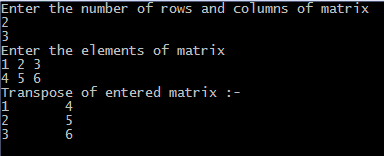## Print this Job Post# C program to transpose a matrix

This c program prints transpose of a matrix. It is obtained by interchanging rows and columns of a matrix. For example if a matrix is
1 2
3 4
5 6
then transpose of above matrix will be
1 3 5
2 4 6
When we transpose a matrix then the order of matrix changes, but for a square matrix order remains same.

## C programming code

#include <stdio.h>
#include<conio.h>
void main()
{
int m, n, c, d, matrix, transpose;
clrscr();
printf("Enter the number of rows and columns of matrix ");
scanf("%d%d",&m,&n);
printf("Enter the elements of matrix \n");
for( c = 0 ; c < m ; c++ )
{
for( d = 0 ; d < n ; d++ )
{
scanf("%d",&matrix[c][d]);
}
}
for( c = 0 ; c < m ; c++ )
{
for( d = 0 ; d < n ; d++ )
{
transpose[d][c] = matrix[c][d];
}
}
printf("Transpose of entered matrix :-\n");
for( c = 0 ; c < n ; c++ )
{
for( d = 0 ; d < m ; d++ )
{
printf("%d\t",transpose[c][d]);
}
printf("\n");
}
getch();
}

## Output of program: